##### 亲爱的游客，欢迎！

•客服电话点击联系客服

在线时间：8:00-16:00

400-123-4567

#### 电子邮件

•APP下载

星点互联APP

随时随地掌握行业动态•官方微信

扫描二维码

关注星点互联微信公众号2019-1-1 12:30

• # 0XFF 前言&概念

-----quhengyi11 dalao

Splay是辅助树，阅读时不要将主的和辅的搞混了。

Link-Cut Tree 准确的说是一个 Splay 森林。每棵 Splay 都用"虚边"链接(下图灰边表示虚边)，每棵 Splay 中的结点都用"实边"链接起来(下图用黑色表示实边)。假如我们现在有一个栗子：(用红色圈圈圈在一起的结点是一个 Splay 中的结点)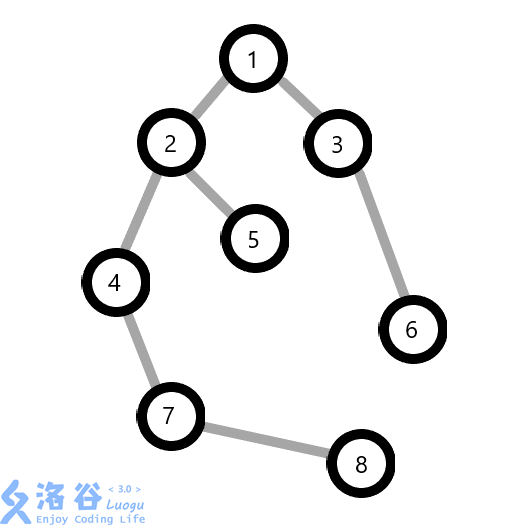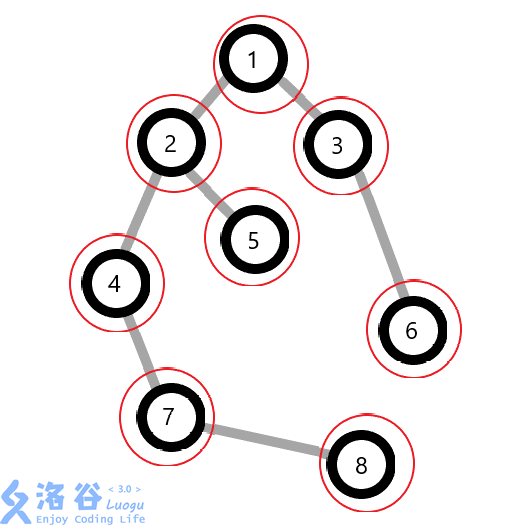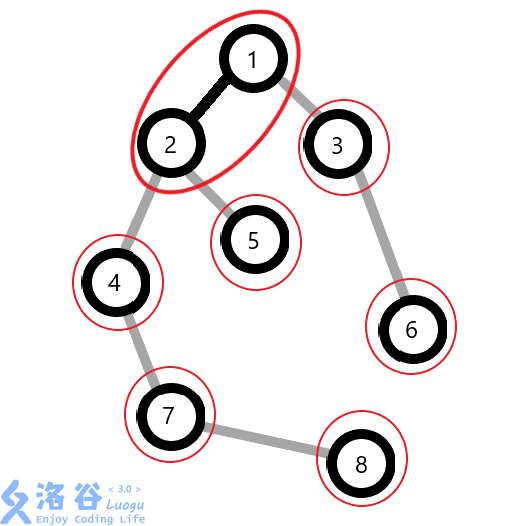• # 0X01 一些基本的定义

`f[x]`:结点x的爸爸(father)

`v[x]`:结点x的权值(value)

`s[x]`:结点x及它的子树的权值和(sum)

`r[x]`:结点x的翻转情况(rev)

`ch[x][0/1]`:结点x的左/右儿子

• # 0X02 一些操作

`Access(x)`：将x到根节点的路径上全部变成实边，并弃掉自己所有的儿子(变成虚边：认父不认子)(每一个父结点对于自己的每个子结点只有一条实边)

`findroot(x)`:找出x所在的原树的根结点(实际上就是上图的一号点)

`makeroot(x)`:这个操作的意思是将x点变为原树的根节点

`split(x,y)`:将x,y搞在一个 Splay 中，以方便操作。

`link(x,y)`:将x和y所在原树合并起来(链接)

`cut(x,y)`:将x和y所在原树拆开(切断)

• # Access(x): 这是最基础的操作，意思是将点x到原树中根结点root之间的链丢到一个辅助树splay里面``````Q：为什么是右儿子而不是左儿子呢？

A：因为f[x]的深度小于x，而在Splay里面f[x]是x的爸爸，所以x在Splay中是f[x]的右儿子。``````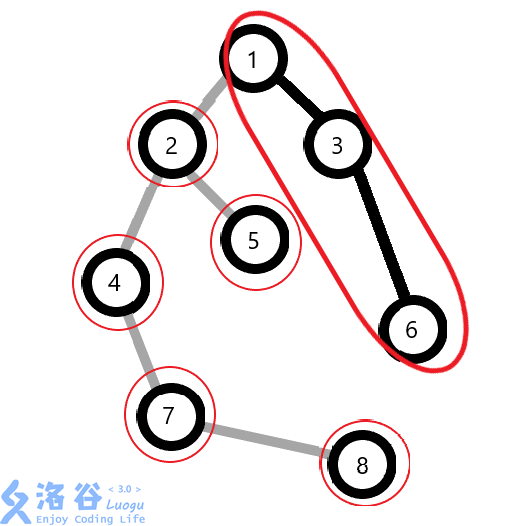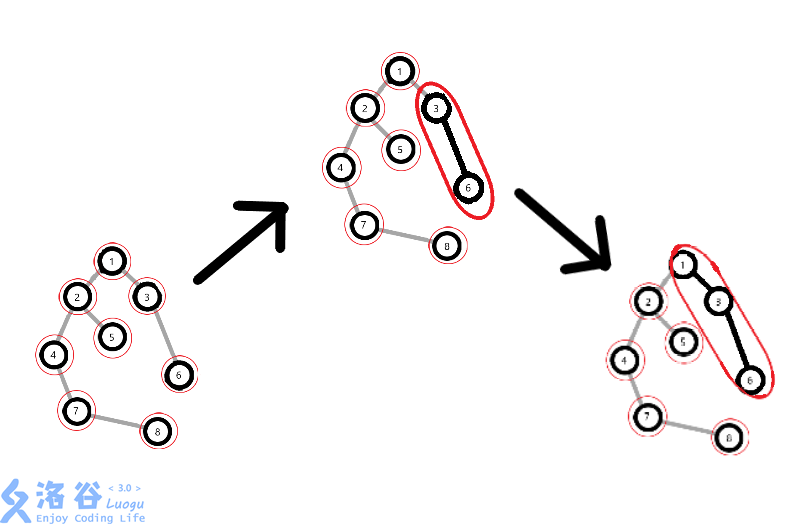1.转到根。

2.换儿子。

3.更新信息。

4.当前操作点切换为轻边所指的父亲，转1。

``````inline void Access(int x){
for(register int y=0;x;y=x,x=f[x]){
Splay(x);//转到所在Splay的根节点
ch[x]=y;//认儿子了
pushup(x);//儿子有变化，更新
}
}``````

• # findroot(x):

• 首先要明白：
• 根节点是的深度最小的

``````inline int findroot(int x){
Access(x);//Access将x和根结点搞到同一个Splay中
Splay(x);//转到Splay的根结点
while(ch[x])pushdown(x),x=ch[x];//不断的找左儿子&更新节点信息
return x;//最左边的就是根结点了。
}``````

• # makeroot(x): 将x到根结点的路径上的点全部翻转(即x变成了根节点)

``````inline void filp(int x){//Splay普通区间翻转
swap(ch[x],ch[x]);r[x]^=1;
}
inline void makeroot(int x){
Access(x);
Splay(x);
filp(x);//懒标记&翻转区间
}``````

• # split(x,y)

Splay 维护的是原树中的一条链，我们不能保证x,y会在同一条链里。

``````inline void split(int x,int y){
makeroot(x);Access(y);Splay(y);
} ``````

``````inline void link(int x,int y){
makeroot(x);//丢到根
if(findroot(y)!=x)f[x]=y;//链接一条虚边
//注意因为是虚边，所以不能认儿子
}``````

• # cut(x,y): 首先我们先把x,y之间的那条边用split(x,y)拎出来，因为x,y是相邻的，所以y的左儿子一定是x，将它们的父子关系消灭掉即可。

1.x和y在一个原树里(不在一个树里面往哪儿切啊)

2.split之后x是y的左儿子

3.x的右儿子是空的(保证了中序遍历中y紧跟在x的后面，即深度相邻)(x的权值(深度)只比y小1，而x又正好是直接连着y的，所以我们无法再找到 >x 而又 <y 的整数了)

``````inline void cut(int x,int y){
split(x,y);
if(findroot(y)==x&&f[x]==y&&!ch[x]){//判断各种条件
f[x]=ch[y]=0;//彻底切断关系
pushup(y);//儿子变了，更新
}return;
}``````

• # 旋转的改动：

## 做个对比：

``````inline void rotate(int x){
int y=f[x],z=f[y],k=chk(x),v=ch[x][!k];
if(get(y))ch[z][chk(y)]=x;ch[x][!k]=y,ch[y][k]=v;
if(v)f[v]=y;f[y]=x,f[x]=z;pushup(y),pushup(x);
}``````

``````inline void rotate(int x){
int y=f[x],z=f[y],k=chk(x),v=ch[x][!k];
ch[z][chk(y)]=x;ch[x][!k]=y,ch[y][k]=v;
f[v]=y;f[y]=x,f[x]=z;pushup(y),pushup(x);
}``````
• # Splay的改动

``````inline void Splay(int x){
int y=x,top=0;hep[++top]=y;
while(get(y))hep[++top]=y=f[y];
while(top)pushdown(hep[top--]);

while(get(x)){//基本普通的Splay
y=f[x],top=f[y];
if(get(y))
rotate((ch[y]==x)^(ch[top]==y)?x:y);
rotate(x);
}pushup(x);return;
}``````

• # 0X04 一些题目代码：

### Code:

``````#include<bits/stdc++.h>
#define ll long long
#define inf 0x3f3f3f3f
#define RI register int
#define A printf("A")
#define C printf(" ")
using namespace std;
const int N=3e5+2;
template <typename Tp> inline void IN(Tp &x){
int f=1;x=0;char ch=getchar();
while(ch<'0'||ch>'9')if(ch=='-')f=-1,ch=getchar();
while(ch>='0'&&ch<='9')x=x*10+ch-'0',ch=getchar();x*=f;
}int f[N],v[N],s[N],r[N],hep[N],ch[N];
inline int get(int x){
return ch[f[x]]==x||ch[f[x]]==x;
}
inline void pushup(int x){
s[x]=s[ch[x]]^s[ch[x]]^v[x];
}
inline void filp(int x){
swap(ch[x],ch[x]);r[x]^=1;
}
inline void pushdown(int x){
if(!r[x])return;r[x]=0;
if(ch[x])filp(ch[x]);
if(ch[x])filp(ch[x]);
}
inline void rotate(int x){
int y=f[x],z=f[y],k=ch[y]==x,v=ch[x][!k];
if(get(y))ch[z][ch[z]==y]=x;ch[x][!k]=y,ch[y][k]=v;
if(v)f[v]=y;f[y]=x,f[x]=z;pushup(y);
}
inline void Splay(int x){
int y=x,top=0;hep[++top]=y;
while(get(y))hep[++top]=y=f[y];
while(top)pushdown(hep[top--]);
while(get(x)){
y=f[x],top=f[y];
if(get(y))
rotate((ch[y]==x)^(ch[top]==y)?x:y);
rotate(x);
}pushup(x);return;
}
inline void Access(int x){
for(register int y=0;x;x=f[y=x])
Splay(x),ch[x]=y,pushup(x);
}
inline void makeroot(int x){
Access(x);Splay(x);filp(x);
}
inline int findroot(int x){
Access(x);Splay(x);
while(ch[x])pushdown(x),x=ch[x];
return x;
}
inline void split(int x,int y){
makeroot(x);Access(y);Splay(y);
}
makeroot(x);if(findroot(y)!=x)f[x]=y;
}
inline void cut(int x,int y){
makeroot(x);
if(findroot(y)==x&&f[x]==y&&!ch[x]){
f[x]=ch[y]=0;pushup(y);
}return;
}int n,m,x,y,op;
int main(){
scanf("%d%d",&n,&m);
for(register int i=1;i<=n;++i)scanf("%d",&v[i]);
for(register int i=1;i<=m;++i){
scanf("%d%d%d",&op,&x,&y);
if(op==0)split(x,y),printf("%d\n",s[y]);
else if(op==2)cut(x,y);
else Splay(x),v[x]=y;
}return 0;
}``````

• ## [SDOI2008]洞穴勘测

### Code:

``````#include<bits/stdc++.h>
#define ll long long
#define inf 0x3f3f3f3f
#define RI register int
#define A printf("A")
#define C printf(" ")
using namespace std;
const int N=2e5+2;
template <typename Tp> inline void IN(Tp &x){
int f=1;x=0;char ch=getchar();
while(ch<'0'||ch>'9')if(ch=='-')f=-1,ch=getchar();
while(ch>='0'&&ch<='9')x=x*10+ch-'0',ch=getchar();x*=f;
}int n,m,f[N],r[N],hep[N],ch[N];
inline int get(int x){return ch[f[x]]==x||ch[f[x]]==x;}
inline void filp(int x){swap(ch[x],ch[x]);r[x]^=1;}
inline void pushdown(int x){
if(!r[x])return;r[x]=0;
if(ch[x])filp(ch[x]);
if(ch[x])filp(ch[x]);
}
inline void rotate(int x){
int y=f[x],z=f[y],k=ch[y]==x,v=ch[x][!k];
if(get(y))ch[z][ch[z]==y]=x;ch[x][!k]=y,ch[y][k]=v;
if(v)f[v]=y;f[y]=x,f[x]=z;return;
}
inline void Splay(int x){
int y=x,top=0;hep[++top]=y;
while(get(y))hep[++top]=y=f[y];
while(top)pushdown(hep[top--]);
while(get(x)){
y=f[x],top=f[y];
if(get(y))
rotate((ch[y]==x)^(ch[top]==y)?x:y);
rotate(x);
}return;
}
inline void Access(int x){
for(register int y=0;x;x=f[y=x])
Splay(x),ch[x]=y;
}
inline void makeroot(int x){
Access(x);Splay(x);filp(x);
}
inline int findroot(int x){
Access(x);Splay(x);
while(ch[x])pushdown(x),x=ch[x];
return x;
}
inline void split(int x,int y){
makeroot(x);Access(y);Splay(y);
}
makeroot(x);if(findroot(y)!=x)f[x]=y;
}
inline void cut(int x,int y){
makeroot(x);
if(findroot(y)==x&&f[x]==y&&!ch[x]){
f[x]=ch[y]=0;
}return;
}char op;
int main(){
scanf("%d%d",&n,&m);
for(register int x,y,i=1;i<=m;++i){
scanf("%s%d%d",op,&x,&y);
else if(op=='D')cut(x,y);
else if(op=='Q'){
if(findroot(x)==findroot(y))printf("Yes\n");
else printf("No\n");
}
}return 0;
}``````

• # 0X05 致谢：

### 全部评论

##### 上一篇：
Django数据库--事务及事务回滚发布时间：2018-12-30
##### 下一篇：
python装饰器(新年第一写)发布时间：2019-01-01